# PySpark – Sort dataframe by multiple columns

• Last Updated : 30 Jun, 2021

In this article, we are going to see how to sort the PySpark dataframe by multiple columns.

It can be done in these ways:

Attention geek! Strengthen your foundations with the Python Programming Foundation Course and learn the basics.

To begin with, your interview preparations Enhance your Data Structures concepts with the Python DS Course. And to begin with your Machine Learning Journey, join the Machine Learning - Basic Level Course

• Using sort()
• Using orderBy()

Creating Dataframe for demonstration:

## Python3

 `# importing module``import` `pyspark`` ` `# importing sparksession from pyspark.sql module``from` `pyspark.sql ``import` `SparkSession`` ` `# creating sparksession and giving an app name``spark ``=` `SparkSession.builder.appName(``'sparkdf'``).getOrCreate()`` ` `# list  of students  data``data ``=` `[[``"1"``, ``"sravan"``, ``"vignan"``],``        ``[``"2"``, ``"ojaswi"``, ``"vvit"``],``        ``[``"3"``, ``"rohith"``, ``"vvit"``],``        ``[``"4"``, ``"sridevi"``, ``"vignan"``],``        ``[``"1"``, ``"sravan"``, ``"vignan"``],``        ``[``"5"``, ``"gnanesh"``, ``"iit"``]]`` ` `# specify column names``columns ``=` `[``'student ID'``, ``'student NAME'``, ``'college'``]`` ` `# creating a dataframe from the lists of data``dataframe ``=` `spark.createDataFrame(data, columns)`` ` `print``(``"Actual data in dataframe"``)`` ` `# show dataframe``dataframe.show()`

Output: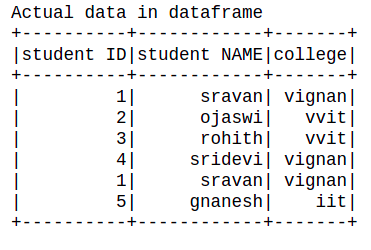### Method 1: Using sort() function

This function is used to sort the column.

Syntax: dataframe.sort([‘column1′,’column2′,’column n’],ascending=True)

Where,

• dataframe is the dataframe name created from the nested lists using pyspark
• where columns are the llst of columns
• ascending = True specifies order the dataframe in increasing order, ascending=False specifies order the dataframe in decreasing order

Example 1: Python code to sort dataframe by passing a list of multiple columns(2 columns) in ascending order.

## Python3

 `# show dataframe by sorting the dataframe``# based on two columns in ascending order``dataframe.sort([``'college'``,``'student ID'``],``               ``ascending ``=` `True``).show()`

Output: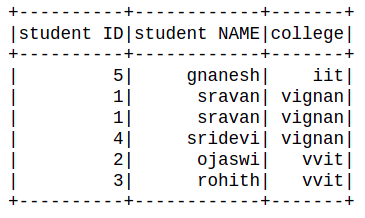Example 2: Python program to sort the data frame by passing a list of columns in descending order

## Python3

 `# show dataframe by sorting the dataframe``# based on two columns in descending order``dataframe.sort([``'college'``,``'student NAME'``],``               ``ascending ``=` `False``).show()`

Output: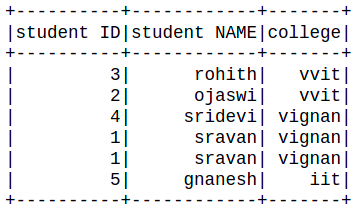### Method 2: Using orderBy() function.

orderBy() function that sorts one or more columns. By default, it orders by ascending.

Syntax: orderBy(*cols, ascending=True)

Parameters:

• cols: Columns by which sorting is needed to be performed.
• ascending: Boolean value to say that sorting is to be done in ascending order

Example 1: Python program to show dataframe by sorting the dataframe based on two columns in descending order using orderby() function

## Python3

 `# show dataframe by sorting the dataframe``# based on two columns in descending``# order using orderby() function``dataframe.orderBy([``'student ID'``,``'student NAME'``],``                  ``ascending ``=` `False``).show()`

Output: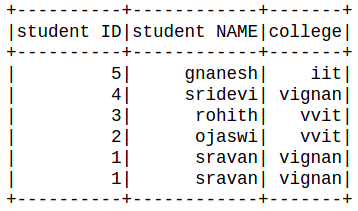Example 2: Python program to show dataframe by sorting the dataframe based on two columns in ascending order using orderby() function

## Python3

 `# show dataframe by sorting the dataframe``# based on two columns in ascending``# order using orderby() function``dataframe.orderBy([``'student ID'``,``'student NAME'``],``                  ``ascending ``=` `True``).show()`

Output: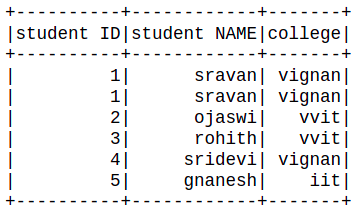My Personal Notes arrow_drop_up